# CBSE Solutions for Class 10 Maths

#### Select CBSE Solutions for class 10 Subject & Chapters Wise :

cos 60° cos 30° − sin 60° sin 30° = ?

Answer :

cos 60o cos 30o − sin 60o sin 30o
=( 1/2 × √3/2 - √3/2 × ½ ) = (√3/4 - √3/4)=0

Evaluate:

sin30°cos45°+cot45°sec60°-sin60°tan45°+cos30°sin90°

Answer :

sin30°cos45°+cot45°sec60°-sin60°tan45°+cos30°sin90°

=(1/2)(1/√2)+1/2-(√3/2)1+(√3/2)1

=√2/2 + ½ - √3/2 + √3/2

=√2+1/2

sin 60° cos 30° + cos 60° sin 30° = ?

Answer :

sin 60o cos 30o + cos 60o sin 30o
=(√3/2 × √3/2 + ½ × ½ ) = (3/4 +1/4 )=4/4=1

cos 45° cos 30° + sin 45° sin 30° = ?

Answer :

cos 45o cos 30o + sin 45o  sin 30o = ?
= (1/√2 × √3/2 + 1/√2 × ½ ) = (√3/2√2 + 1/2√2) = (√3 +1/2√2)

Evaluate:

5cos260°+4sec230°-tan245°sin230°+cos230°

Answer :

5cos260°+4sec230°-tan245°sin230°+cos230°=

5(1/2)2 + 4(2√3)2 -(1)2(1/2)2+(√3/2)2

=(5/4+4×4/3-1)(1/4+3/4)

=(5/4+16/3-1)(4/4)

=[(15+64-12)/12](4/4)

=(67/12)(1)

=67/12

2 cos2 60° + 3 sin2 45° − 3 sin2 30° + 2 cos2 90° = ?

Answer :

2 cos2 60o + 3 sin2 45o − 3 sin2 30o + 2 cos2 90o
=2×(1/2)2+3×(1/√2)2-3×(1/2)2 + 2×(0)2

=2×1/4 + 3×1/2- 3×1/4+0

=(1/2+3/2-3/4)
=[(2+6-3)/4]

=54

cot230° − 2cos230° − 3/4sec245° + 1/4 cosec230° = ?

Answer :

cot2 30o − 2 cos2 30o – 3/4sec2 45o + 1/4cosec230o
= (√3)2-2×(√3/2)2-3/4×(√2)2+1/4×(2)2

= 3-2×3/4-3/4×2+1/4×4

= 3-3/2-3/2+1

= 4-(3/2+3/2)

= 4-3= 1

(sin230° + 4cot245° − sec260°)(cosec245° sec230°) = ?

Answer :

(sin2 30o + 4 cot2 45o − sec2 60o )(cosec2 45sec2 30o)
=[(1/2)2+4×(1)2-(2)2] [(√2)2 (2/√3)2]=(1/4+4-4) (2×4/3)

=1/4×8/3=2/3

4/cot230°+1/sin230°-2cos245°-sin2 = ?

Answer :

4cot230° +1sin230° -2 cos245o -sin20o

=4(√3)2+1(1/2)2 - 2×(1/√2)2-(0)2

=4/3+1/4-2×1/2-0

=4/3+4-1 =4/3+3

=(4+9)/3 = 13/3

(1-sin60°)/cos60°= (tan60°-1)/tan60°+1

Answer :

LHS=1-sin 60ocos 60o=1-√3212=(2-√32)12=(2-√32)×2=2-√3RHS= tan 60o-1tan 60o+1=√3-1√3+1=√3-1√3+1×√3 -1√3 -1=(√3-1)2(√3)2-12=3+1-2√33-1=4-2√32=2-√3

Hence, LHS = RHS

1-sin 60ocos 60o=tan 60o-1tan 60o+1

If sinθ = cos(θ-45°), where θ is acute, then find the value of θ.

Answer :

We have, sinθ=cos(θ-45°)

cos(90°-θ)=cos(θ-45°)

Comparing both sides, we get

90°- θ = θ - 45°

θ+θ = 90°+45°

2θ=135°

θ=(135/2)°

θ=67.5°

If tanA=5/12, then find the value of (sinA+cosA)secA.                [CBSE 2008]

Answer :

(sinA+cosA)secA=(sinA+cosA)/cosA=sinA/cosA+cosA/cosA=tanA+1= 5/12+1/1 =(5+12)/12 = 17/12

Write the value of cos1° cos2° ... cos180°.

Answer :

cos1° cos2° ... cos180°=cos1° cos2° ... cos90° ... cos180°=cos1° cos2° ... 0 ... cos180°=0

Find the value of sin50°/cos40° + cosec40°/sec50°- 4cos50° cosec40°.

Answer :

sin50°/cos40° + cosec40°/sec50° -4cos50° cosec40° = cos(90°-50°)/cos40° + sec(90°-40°)/sec50° - 4sin(90°-50°) cosec40°

=cos40°/cos40° + sec50°/sec50° - 4sin40°/sin40° = 1+1 – 4 = -2

Find the value of sin48° sec42°+cos48° cosec42°.

Answer :

sin48° sec42°+cos48° cosec42°= sin48° cosec(90°-42°) + cos48° sec(90°-42°) =sin48° cosec48°+cos48° sec48° =sin48°/sin48° + cos48°/cos48° =1+1 = 2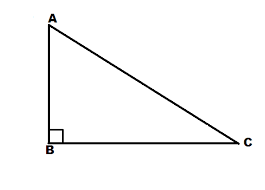1 Sin A A AB/AC 2 Cos A B BC/AC 3 Tan A C BC/AB

Answer :

1-B, 2-A, 3-C1 Cot A A AC/AB 2 Cosec A B AB/BC 3 Sec A C AC/BC

Answer :

1-B, 2-C, 3-A

 1 Sin 0° A 32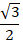2 Sin 30° B 0 3 Sin 45° C ½ 4 Sin 60° D 1/√2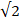Answer :

1-B, 2-C, 3-D, 4-A

 1 Sin θ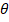A Perpendicular ÷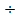Base 2 Cos θB Perpendicular ÷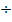Hypotenuse 3 Tan θC Base ÷Hypotenuse

Answer :

1-B, 2-C, 3-A

 1 Cos 0° A ½ 2 Cos 30° B 1/√23 Cos 45° C 32.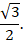4 Cos 60° D 1

Answer :

1-D, 2-C, 3-B, 4-A

### Take a Test

Choose your Test :

### Chapter 8 : Introduction to Trigonometry

You have already studied about triangles, and in particular, right triangles, in your earlier classes. In this chapter, we will study some ratios of the sides of a right triangle with respect to its acute angles, called trigonometric ratios of the angle. We also define the trigonometric ratios for angles of measure 0o and 90o. We will calculate trigonometric ratios for some specific angles and establish some identities involving these ratios, called trigonometric identities.

### Browse & Download CBSE Books For Class 10 - All Subjects

The GSEB Books for class 10 are designed as per the syllabus followed Gujarat Secondary and Higher Secondary Education Board provides key detailed, and a through solutions to all the questions relating to the GSEB textbooks.

The purpose is to provide help to the students with their homework, preparing for the examinations and personal learning. These books are very helpful for the preparation of examination.

For more details about the GSEB books for Class 10, you can access the PDF which is as in the above given links for the same.